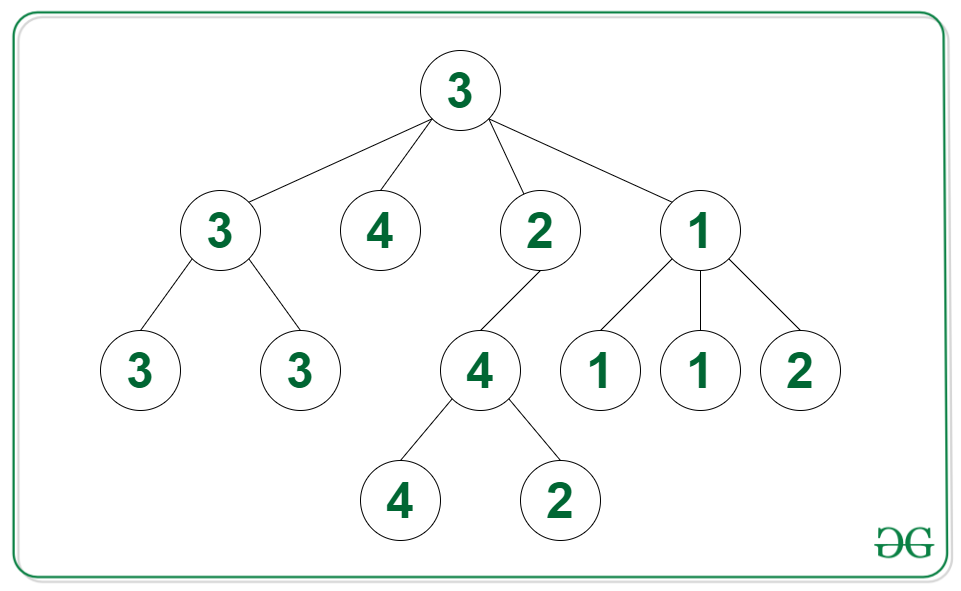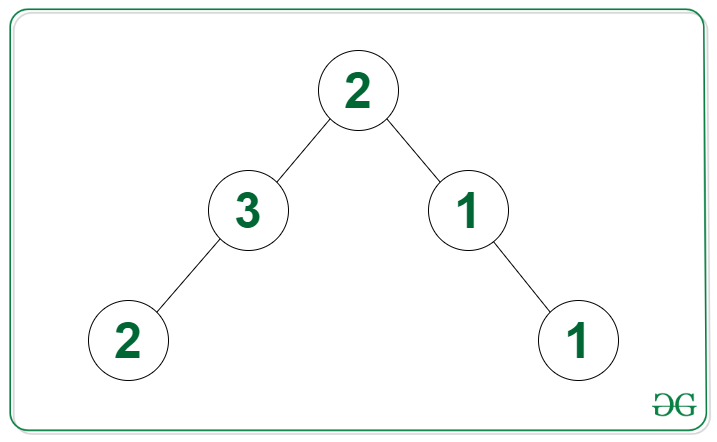GeeksforGeeks App
Open AppBrowser
Continue

# Maximum count of connected duplicate nodes in given N-ary Tree

Given a generic tree such that each node has a value associated with it, the task is to find the largest number of connected nodes having the same value in the tree. Two nodes are connected if one node is a child of another node.

Example:

Input: Tree in the image belowOutput: 4
Explanation: The largest group of connected nodes are of the value 3 with number of nodes equal to 4.

Input: Tree in the image belowOutput: 2

Approach: The given problem can be solved by using the post-order traversal. The idea is to check if the child node has the same value as its parent node and add 1 to the answer returned from the child node. Below steps can be followed to solve the problem:

• Apply post-order traversal on the N-ary tree:
• If the root has no children then return 1 to the parent
• Add all the answers returned from the children nodes whose value is same as current node
• Update the maximum number of connected nodes
• Return the maximum number of connected nodes as the answer

Below is the implementation of the above approach:

## C++

 `// C++ code for the above approach``#include ``using` `namespace` `std;` `class` `Node {``public``:``    ``vector children;``    ``int` `val;` `    ``// constructor``    ``Node(``int` `v)``    ``{` `        ``val = v;``        ``children = {};``    ``}``};``// Post order traversal function``// to calculate the largest group``// of connected nodes``int` `postOrder(Node* root, ``int` `maxi[])``{` `    ``// If the current node has no``    ``// children then return 1``    ``if` `(root->children.size() == 0)``        ``return` `1;` `    ``// Initialize a variable sum to``    ``// calculate largest group connected``    ``// to current node with same value``    ``// as current node``    ``int` `sum = 1;` `    ``// Iterate through all neighbors``    ``for` `(Node* child : root->children) {` `        ``// Get the value from children``        ``int` `nodes = postOrder(child, maxi);` `        ``// If child node value is same as``        ``// current node then add the``        ``// returned value to sum``        ``if` `(child->val == root->val)``            ``sum += nodes;``    ``}` `    ``// Update maximum connected``    ``// nodes if sum is greater``    ``maxi = max(maxi, sum);` `    ``// Return the connected group``    ``// to the current node``    ``return` `sum;``}``// Function to find the largest``// number of nodes in a tree``int` `largestGroup(Node* root)``{` `    ``// Base case``    ``if` `(root == NULL)``        ``return` `0;` `    ``// Initialize a variable max``    ``// to calculate largest group``    ``int` `maxi;` `    ``// Post-order traversal``    ``postOrder(root, maxi);` `    ``// Return the answer``    ``return` `maxi;``}` `// Driver code``int` `main()``{` `    ``// Initialize the tree``    ``Node* three1 = ``new` `Node(3);``    ``Node* three2 = ``new` `Node(3);``    ``Node* three3 = ``new` `Node(3);``    ``Node* three4 = ``new` `Node(3);``    ``Node* two1 = ``new` `Node(2);``    ``Node* two2 = ``new` `Node(2);``    ``Node* two3 = ``new` `Node(2);``    ``Node* two4 = ``new` `Node(2);``    ``Node* four1 = ``new` `Node(4);``    ``Node* four2 = ``new` `Node(4);``    ``Node* four3 = ``new` `Node(4);``    ``Node* one1 = ``new` `Node(1);``    ``Node* one2 = ``new` `Node(1);``    ``Node* one3 = ``new` `Node(1);``    ``Node* one4 = ``new` `Node(1);``    ``three2->children.push_back(two1);``    ``three2->children.push_back(three1);``    ``three2->children.push_back(three3);``    ``four1->children.push_back(four2);``    ``four1->children.push_back(four3);``    ``two2->children.push_back(one1);``    ``two2->children.push_back(one2);``    ``two2->children.push_back(two3);``    ``one3->children.push_back(one4);``    ``one3->children.push_back(two4);``    ``three4->children.push_back(three2);``    ``three4->children.push_back(four1);``    ``three4->children.push_back(two2);``    ``three4->children.push_back(one3);` `    ``// Call the function``    ``// and print the result``    ``cout << (largestGroup(three4));``}` `// This code is contributed by Potta Lokesh`

## Java

 `// Java implementation for the above approach` `import` `java.io.*;``import` `java.util.*;` `class` `GFG {` `    ``static` `class` `Node {` `        ``List children;``        ``int` `val;` `        ``// constructor``        ``public` `Node(``int` `val)``        ``{` `            ``this``.val = val;``            ``children = ``new` `ArrayList<>();``        ``}``    ``}` `    ``// Function to find the largest``    ``// number of nodes in a tree``    ``public` `static` `int` `largestGroup(Node root)``    ``{` `        ``// Base case``        ``if` `(root == ``null``)``            ``return` `0``;` `        ``// Initialize a variable max``        ``// to calculate largest group``        ``int``[] max = ``new` `int``[``1``];` `        ``// Post-order traversal``        ``postOrder(root, max);` `        ``// Return the answer``        ``return` `max[``0``];``    ``}` `    ``// Post order traversal function``    ``// to calculate the largest group``    ``// of connected nodes``    ``public` `static` `int` `postOrder(``        ``Node root, ``int``[] max)``    ``{` `        ``// If the current node has no``        ``// children then return 1``        ``if` `(root.children.size() == ``0``)``            ``return` `1``;` `        ``// Initialize a variable sum to``        ``// calculate largest group connected``        ``// to current node with same value``        ``// as current node``        ``int` `sum = ``1``;` `        ``// Iterate through all neighbors``        ``for` `(Node child : root.children) {` `            ``// Get the value from children``            ``int` `nodes = postOrder(child, max);` `            ``// If child node value is same as``            ``// current node then add the``            ``// returned value to sum``            ``if` `(child.val == root.val)``                ``sum += nodes;``        ``}` `        ``// Update maximum connected``        ``// nodes if sum is greater``        ``max[``0``] = Math.max(max[``0``], sum);` `        ``// Return the connected group``        ``// to the current node``        ``return` `sum;``    ``}` `    ``// Driver code``    ``public` `static` `void` `main(String[] args)``    ``{` `        ``// Initialize the tree``        ``Node three1 = ``new` `Node(``3``);``        ``Node three2 = ``new` `Node(``3``);``        ``Node three3 = ``new` `Node(``3``);``        ``Node three4 = ``new` `Node(``3``);``        ``Node two1 = ``new` `Node(``2``);``        ``Node two2 = ``new` `Node(``2``);``        ``Node two3 = ``new` `Node(``2``);``        ``Node two4 = ``new` `Node(``2``);``        ``Node four1 = ``new` `Node(``4``);``        ``Node four2 = ``new` `Node(``4``);``        ``Node four3 = ``new` `Node(``4``);``        ``Node one1 = ``new` `Node(``1``);``        ``Node one2 = ``new` `Node(``1``);``        ``Node one3 = ``new` `Node(``1``);``        ``Node one4 = ``new` `Node(``1``);``        ``three2.children.add(two1);``        ``three2.children.add(three1);``        ``three2.children.add(three3);``        ``four1.children.add(four2);``        ``four1.children.add(four3);``        ``two2.children.add(one1);``        ``two2.children.add(one2);``        ``two2.children.add(two3);``        ``one3.children.add(one4);``        ``one3.children.add(two4);``        ``three4.children.add(three2);``        ``three4.children.add(four1);``        ``three4.children.add(two2);``        ``three4.children.add(one3);` `        ``// Call the function``        ``// and print the result``        ``System.out.println(``            ``largestGroup(three4));``    ``}``}`

## Python3

 `# Python code for the above approach``class` `Node:` `  ``# constructor``  ``def` `__init__(``self``, v):``    ``self``.val ``=` `v;``    ``self``.children ``=` `[];``  ` `# Post order traversal function``# to calculate the largest group``# of connected nodes``def` `postOrder(root, maxi):` `  ``# If the current node has no``  ``# children then return 1``  ``if` `(``len``(root.children) ``=``=` `0``):``    ``return` `1``;` `  ``# Initialize a variable sum to``  ``# calculate largest group connected``  ``# to current node with same value``  ``# as current node``  ``sum` `=` `1``;` `  ``# Iterate through all neighbors``  ``for` `child ``in` `root.children:` `    ``# Get the value from children``    ``nodes ``=` `postOrder(child, maxi);` `    ``# If child node value is same as``    ``# current node then add the``    ``# returned value to sum``    ``if` `(child.val ``=``=` `root.val):``      ``sum` `+``=` `nodes;``  ` `  ``# Update maximum connected``  ``# nodes if sum is greater``  ``maxi[``0``] ``=` `max``(maxi[``0``], ``sum``);` `  ``# Return the connected group``  ``# to the current node``  ``return` `sum``;` `# Function to find the largest``# number of nodes in a tree``def` `largestGroup(root):` `  ``# Base case``  ``if` `(root ``=``=` `None``):``    ``return` `0``;` `  ``# Initialize a variable max``  ``# to calculate largest group``  ``maxi ``=` `[``0``];` `  ``# Post-order traversal``  ``postOrder(root, maxi);` `  ``# Return the answer``  ``return` `maxi[``0``];`  `# Driver code` `# Initialize the tree``three1 ``=` `Node(``3``);``three2 ``=` `Node(``3``);``three3 ``=` `Node(``3``);``three4 ``=` `Node(``3``);``two1 ``=` `Node(``2``);``two2 ``=` `Node(``2``);``two3 ``=` `Node(``2``);``two4 ``=` `Node(``2``);``four1 ``=` `Node(``4``);``four2 ``=` `Node(``4``);``four3 ``=` `Node(``4``);``one1 ``=` `Node(``1``);``one2 ``=` `Node(``1``);``one3 ``=` `Node(``1``);``one4 ``=` `Node(``1``);``three2.children.append(two1);``three2.children.append(three1);``three2.children.append(three3);``four1.children.append(four2);``four1.children.append(four3);``two2.children.append(one1);``two2.children.append(one2);``two2.children.append(two3);``one3.children.append(one4);``one3.children.append(two4);``three4.children.append(three2);``three4.children.append(four1);``three4.children.append(two2);``three4.children.append(one3);` `# Call the function``# and print the result``print``((largestGroup(three4)));` `# This code is contributed by gfgking`

## C#

 `// C# implementation for the above approach``using` `System;``using` `System.Collections.Generic;`  `// Class representing a Node of an N-ary tree``public` `class` `Node``{``  ``public` `int` `val;``  ``public` `List children;` `  ``// Constructor to create a Node``  ``public` `Node(``int` `vall)``  ``{``    ``val = vall;``    ``children = ``new` `List();``  ``}``}` `class` `GFG {` `  ``// Function to find the largest``  ``// number of nodes in a tree``  ``public` `static` `int` `largestGroup(Node root)``  ``{` `    ``// Base case``    ``if` `(root == ``null``)``      ``return` `0;` `    ``// Initialize a variable max``    ``// to calculate largest group``    ``int``[] max = ``new` `int``;` `    ``// Post-order traversal``    ``postOrder(root, max);` `    ``// Return the answer``    ``return` `max;``  ``}` `  ``// Post order traversal function``  ``// to calculate the largest group``  ``// of connected nodes``  ``public` `static` `int` `postOrder(``    ``Node root, ``int``[] max)``  ``{` `    ``// If the current node has no``    ``// children then return 1``    ``if` `(root.children.Count == 0)``      ``return` `1;` `    ``// Initialize a variable sum to``    ``// calculate largest group connected``    ``// to current node with same value``    ``// as current node``    ``int` `sum = 1;` `    ``// Iterate through all neighbors``    ``foreach` `(Node child ``in` `root.children) {` `      ``// Get the value from children``      ``int` `nodes = postOrder(child, max);` `      ``// If child node value is same as``      ``// current node then Add the``      ``// returned value to sum``      ``if` `(child.val == root.val)``        ``sum += nodes;``    ``}` `    ``// Update maximum connected``    ``// nodes if sum is greater``    ``max = Math.Max(max, sum);` `    ``// Return the connected group``    ``// to the current node``    ``return` `sum;``  ``}` `  ``// Driver code``  ``static` `public` `void` `Main (){` `    ``// Initialize the tree``    ``Node three1 = ``new` `Node(3);``    ``Node three2 = ``new` `Node(3);``    ``Node three3 = ``new` `Node(3);``    ``Node three4 = ``new` `Node(3);``    ``Node two1 = ``new` `Node(2);``    ``Node two2 = ``new` `Node(2);``    ``Node two3 = ``new` `Node(2);``    ``Node two4 = ``new` `Node(2);``    ``Node four1 = ``new` `Node(4);``    ``Node four2 = ``new` `Node(4);``    ``Node four3 = ``new` `Node(4);``    ``Node one1 = ``new` `Node(1);``    ``Node one2 = ``new` `Node(1);``    ``Node one3 = ``new` `Node(1);``    ``Node one4 = ``new` `Node(1);``    ``three2.children.Add(two1);``    ``three2.children.Add(three1);``    ``three2.children.Add(three3);``    ``four1.children.Add(four2);``    ``four1.children.Add(four3);``    ``two2.children.Add(one1);``    ``two2.children.Add(one2);``    ``two2.children.Add(two3);``    ``one3.children.Add(one4);``    ``one3.children.Add(two4);``    ``three4.children.Add(three2);``    ``three4.children.Add(four1);``    ``three4.children.Add(two2);``    ``three4.children.Add(one3);` `    ``// Call the function``    ``// and print the result``    ``Console.WriteLine(``      ``largestGroup(three4));``  ``}``}` `// This code is contributed``// by Shubham Singh`

## Javascript

 ``

Output:

`4`

Time Complexity: O(N), where N is the number of nodes in the tree
Auxiliary Space: O(H), H is the height of the tree

My Personal Notes arrow_drop_up